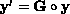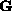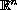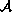Electron. J. Diff. Eqns., Vol. 2005(2005), No. 40, pp. 1-17.

### Some properties of solutions to polynomial systems of differential equations David C. Carothers, G. Edgar Parker, James S. Sochacki, Paul G. Warne

Abstract:
In  and , Parker and Sochacki considered iterative methods for computing the power series solution towhereis a polynomial fromto, including truncations of Picard iteration. The authors demonstrated that many ODE's may be transformed into computationally feasible polynomial problems of this type, and the methods generalize to a broad class of initial value PDE's. In this paper we show that the subset of the real analytic functionsconsisting of functions that are components of the solution to polynomial differential equations is a proper subset ofand that it shares the field and near-field structure of, thus making it a proper sub-algebra. Consequences of the algebraic structure are investigated. Using these results we show that the Maclaurin or Taylor series can be generated algebraically for a large class of functions. This finding can be used to generate efficient numerical methods of arbitrary order (accuracy) for initial value ordinary differential equations. Examples to indicate these techniques are presented. Future advances in numerical solutions to initial value ordinary differential equations are indicated.

Submitted August 26, 2004. Published April 5, 2005.
Math Subject Classifications: 34A05, 34A25, 34A34, 65L05.
Key Words: Analytic functions; inverse functions; Maclaurin polynomials; Pade expansions; Grobner bases.

Show me the PDF file (253K), TEX file, and other files for this article.David C. Carothers Department of Mathematics and Statistics James Madison University Harrisonburg, VA 22807, USA email: carothdc@jmu.edu G. Edgar Parker Department of Mathematics and Statistics James Madison University Harrisonburg, VA 22807, USA email: parkerge@jmu.edu James S. Sochacki Department of Mathematics and Statistics James Madison University Harrisonburg, VA 22807, USA email: jim@math.jmu.edu Paul G. Warne Department of Mathematics and Statistics James Madison University Harrisonburg, VA 22807, USA email: warnepg@math.jmu.edu